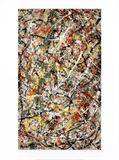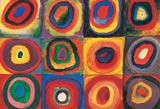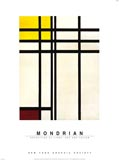## CS 124, Fall 2009 Lab 5: Art(?)

This lab helps your consolidate your understanding of control structures by asking you to use for loops, nested loops, and either a switch statement or an if..else if statement. You will do this in the context of drawing random pictures that resemble abstract art. (If you are getting tired of randomness, I apologize.) The program that you will write will draw a new random art work every five seconds. Here is my program, running as an applet version:

As usual, you should create a lab5 folder in your cs124 directory. As a starting point for your work, you will need a copy of the file RandomArt.java, which you can copy from /classes/f09/cs124.

The random art program is the only exercise for this lab. It is due next Thursday, at the start of next week's lab, as usual.

### New Graphics Commands

You can, of course, use all the graphics commands that were described in Lab 2 such as g.drawLine, g.fillRect, and g.drawString(). Here are three additional, somewhat less commonly used routines:

• `g.fill3DRect(x,y,width,height,raised)` -- Draws a filled-in rectangle that has a slight "three-dimensional" effect that makes it look like it is pushed in or pulled out a bit from the plane of the screen. The upper left corner of the rectangle is at the point (x,y). The width and height are positive integers giving the size of the rectangle, measured in pixels. The fifth parameter, raised, is a boolean value, that is, it can be either true or false. A true value is supposed to make the rectangle look like it is raised above the screen, while a false value is supposed to make it look like it is depressed into the screen. Usually, you should use true for the last parameter.
• `g.fillRoundRect(x,y,width,height,hCornerRadius,vCornerRadius)` -- Draws a filled rectangle, similar to that drawn by g.fillRect(x,y,width,height), except that the corners are rounded. A little quarter-oval is cut off of each corner. The hCornerRadius and vCornerRadius parameters give the horizontal and vertical radii of the oval, so that larger values of these parameters give more rounded corners.
• `g.drawRoundRect(x,y,width,height,hCornerRadius,vCornerRadius)` -- Same as g.fillRoundRect, but only draws the outline of the rounded rectangle.

RandomArtPanel.java defines two useful functions, randomHue(s,b) and randomColor(), which can be used for creating random colors. (Remember that a function is a subroutine that returns a value. In Chapter 4, you will finally learn how to write your own functions, but you already know how to call functions that someone else has defined.) The randomColor() function takes no parameters and simply creates a color with random amounts of red, green, and blue. You would use it in a statement such as "g.setColor(randomColor());". I use this method for the background color in the sample artwork in RandomArt.java. The randomHue() function uses a different way of specifying colors, in terms of "hue," "saturation," and "brightness." It takes two parameters, representing the saturation and brightness of the color, and it uses a random hue. The saturation and the brightness must be real numbers in the range 0 to 1. (You can probably best understand these terms by experimenting with the function.) Here are some examples of using this function:

• `g.setColor(randomHue(1, 1))` -- sets the drawing color to be a bright, fully saturated random color. I use this for the first rectangle color in RandomArt.java.
• `g.setColor(randomHue(1, 0.5))` -- sets the drawing color to be a saturated but rather dull random color.
• `g.setColor(randomHue(0.5, 1))` -- sets the drawing color to a bright, but unsaturated color.
• `g.setColor(randomHue(0,Math.random()))` -- sets the drawing color to to a random shade of gray.

### Random Art

Your assignment for this lab is to create at least four different types of random "art." You should start with the file RandomArt.java. You will do all your work within the paintComponent() method. In the starting file, the paintComponent() method simply draws four random-hued rectangles on a randomly colored background.

You could begin by setting up an overall outline for the paintComponent() method so that it has a random chance of doing any of four different things, with an equal chance for each possibility. It is probably easiest to do this with a switch statement, but you could also use an if..else if statement. You then just have to work on each case of the switch statement separately. Note that you can assume that the drawing area is 500 pixels wide and 400 pixels high (or you can change the size if you want).Case 1: Pseudo Pollack. For your first type of art, you are required to use a for loop to draw a large number of lines. Jackson Pollock is known for large canvases covered with splashes and dribbles of paint. To get something that is vaguely in the Pollack style, you can just draw a large number of random lines of different colors. My version also selects the number of lines at random. It draws bright, unsaturated lines on a white background. You are not required to draw exactly the same type of art as my sample. You might, for example, draw random-length horizontal lines instead. Here is some sample code that will draw a random line on a 500-by-400 drawing area:

```int x1, y1, x2, y2;
x1 = (int)(500*Math.random());
y1 = (int)(400*Math.random());
x2 = (int)(500*Math.random());
y2 = (int)(400*Math.random());
g.drawLine(x1,y1,x2,y2);
```Case 2: Kan't be Kandinsky. Some of the canvases by Wassily Kandinsky feature a grid of repetitive patterns in different colors. To imitate this style, you should cover the drawing area with small squares. Each square should show a similar pattern, but the colors should vary randomly. In my version, I draw 50-by-50 pixel squares. I fill each square with a random background color, then draw a 40-by-40 pixel 3DRect in the center of the square, also in a random color. You don't have to follow this pattern exactly, but you should do some kind of repetitive pattern. You are required to use nested for loops for this case. (Actually, Kandinsky isn't the right model for this part -- there are other artists who do much more regular grids that are more similar to what my program does, but I couldn't remember their names. Does anyone know?)Case 3: Mangled Mondrian. Many paintings by Piet Mondrian show rectangular areas bounded by vertical and horizontal bars. My Mondrian-like artworks show randomly colored vertical and horizontal bars on a randomly colored background; they are based on an exercise used in CS124 by Professor John Vaughn a few years ago. In these drawings, to draw one of the bars, I first decide randomly whether to make it horizontal or vertical. For a horizontal bar, I use g.fill3DRect(0,y,width,h,true), where h is a randomly chosen integer less than 15 that gives the thickness of the bar, and y is a randomly chosen integer less than height that gives the location of the bar. Vertical bars are similar, using g.fill3DRect(x,0,w,height,true). (Here, width and height are just the width and height of the drawing area.) I also select the number of bars at random. For Case 3, you should try to produce something that is as similar to my version as possible.

Case 4: Go Wild For the fourth type of art, you can do whatever you like. Try to be creative. (One idea is to sprinkle a lot of things at random locations all over the canvas. Don't forget that you can use strings as well as shapes.)